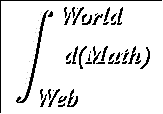#Calculus Index

Paths: Topics:
• Summary
• Limits

• Differentiation
• Table of derivatives
• Definition of differentiation
• The Notation of Differentiation
• Rules of Differentiation
• Derivatives of Trigonometric functions
• Derivative of sine
• Derivatives of cosine
• Derivatives of other trigonometric functions
• Derivatives of inverse trigonometric functions
• Derivatives of Logarithmic Functions
• Euler's magic number/ Derivative of exponentials
• Differentiation of logarithms
• Derivatives of hyperbolic functions

• Applications of Differentiation
• Integration
• Methods of integration

Back to the World Web Math Home Page | Back to the World Web Math Categories Page

watko@mit.edu